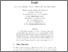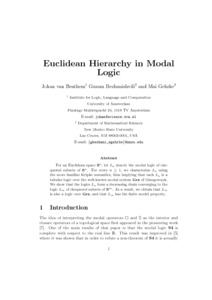# PP-2002-07: Euclidean Hierarchy in Modal Logic

PP-2002-07: van Benthem, Johan and Bezhanishvili, Guram and Gehrke, Mai (2002) Euclidean Hierarchy in Modal Logic. [Report]Preview Text (Full Text) PP-2002-07.text.pdf Download (190kB) | PreviewText (Abstract) PP-2002-07.abstract.txt Download (724B)

## Abstract

For an Euclidean space $\mathbb{R}^n$, let $L_n$ denote the modal
logic of chequered subsets of $\mathbb{R}^n$. For every $n\geq 1$, we
characterize $L_n$ using the more familiar Kripke semantics, thus
implying that each $L_n$ is a tabular logic over the well-known modal
system Grz of Grzegorczyk. We show that the logics $L_n$ form a
decreasing chain converging to the logic $L_\infty$ of chequered
subsets of $\mathbb{R}^\infty$. As a result, we obtain that $L_\infty$
is also a logic over Grz, and that $L_\infty$ has the finite model
property.

Item Type: Report PP-2002-07 Prepublication (PP) Series 2002 Topo-bisimulation, serial set, chequered set, Euclidean hierarchy. 12 Oct 2016 14:36 12 Oct 2016 14:36 https://eprints.illc.uva.nl/id/eprint/71View Item